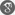##### Cite as:
B.M.B. Krushna, K.R. Prasad, On Existence of Solutions to the Caputo Type Fractional Order Three-Point Boundary Value Problems, Int. J. Anal. Appl., 12 (2) (2016), 80-86.

#### Abstract

In this paper, we establish the existence of solutions to the fractional order three-point boundary value problems by utilizing Banach contraction principle and Schaefer's fixed point theorem.

#### References

1. Z. Bai and Y. Zhang, Solvability of fractional three-point boundary value problems with nonlinear growth, Appl. Math. Comput., 218(2011), 1719–1725.2. M. Benchohra, J. Henderson, S. K. Ntoyuas and A. Ouahab, Existence results for fractional order functional differential equations with infinite delay, J. Math. Anal. Appl., 338 (2008), 1340–1350.3. M. Benchohra, S. Hamani, S.K. Ntouyas, Boundary value problems for differential equations with fractional order and nonlocal conditions, Nonlinear Anal. TMA., 71(2009), 2391–2396.4. M. Feng, X. Zhang and W. Ge, New existence results for higher-order nonlinear fractional differential equation with integral boundary conditions, Bound. Value Probl., 2011(2011), Article ID 720702.5. A. A. Kilbas and J. J. Trujillo, Differential equations of fractional order methods, results, problems, Appl. Anal., 78(2001), 153–192.6. A. A. Kilbas, H. M. Srivasthava and J. J. Trujillo, Theory and Applications of Fractional Differential Equations, North-Holland Mathematics Studies, 204, Elsevier Science, Amsterdam, 2006.7. R. A. Khan, M. Rehman and J. Henderson, Existence and uniqueness of solutions for nonlinear fractional differential equations with integral boundary conditions, Fract. Differ. Calc., 1(2011), 29–43.8. R. A. Khan and H. Khan, Existence of solution for a three-point boundary value problem of fractional differential equation, Journal of Fractional Calculus and Applications, 5(2014), 156–164.9. K. S. Miller and B. Ross, An Introduction to Fractional Calculus and Fractional Differential Equations, John Wiley and Sons, New York, 1993.10. Z. Ouyang and G. Li, Existence of the solutions for a class of nonlinear fractional order three-point boundary value problems with resonance, Bound. Value Probl., 2012(2012), Article ID 68, 1–13.11. I. Podulbny, Fractional Diffrential Equations, Academic Press, San Diego, 1999.12. K. R. Prasad and B. M. B. Krushna, Multiple positive solutions for a coupled system of Riemann–Liouville fractional order two-point boundary value problems, Nonlinear Stud., 20(2013), 501–511.13. K. R. Prasad and B. M. B. Krushna, Eigenvalues for iterative systems of Sturm–Liouville fractional order two-point boundary value problems, Fract. Calc. Appl. Anal., 17(2014), 638–653.14. K. R. Prasad and B. M. B. Krushna, Existence of solutions for a coupled system of three-point fractional order boundary value problems, Differ. Equ. Appl., 7(2015), 187–200.15. M. Rehman and R. A. Khan, Existence and uniqueness of solutions for multi-point boundary value problems for fractional differential equations, Appl. Math. Lett., 23 (2010), 1038–1044.16. M. Rehman, R. A. Khan and N. A. Asif, Three point boundary value problems for nonlinear fractional differential equations, Acta Mathematica Scientia, 31(2011), 1337–1346.17. A. Shi and S. Zhang, Upper and lower solutions method and a fractional differential equation boundary value problem, Electron. J. Qual. Theory Differ. Equ., 30(2009), 1–13.18. G. Wang, S. K. Ntouyas and L. Zhang, Positive solutions of the three-point boundary value problem for fractional order differential equations with an advanced argument, Advances in Difference Equations, 2011(2011), Article ID 2.19. J. R. Wang, L. Lv and W. Wei, Differential equations of fractional order α ∈ (2,3) with boundary value conditions in abstract Banach spaces, Math. Commun., 17(2012), 371–387.20. S. Zhang, Positive solutions for boundary value problems of nonlinear fractional differential equations, Electron. J. Differential Equations, 36(2006), 1–12.